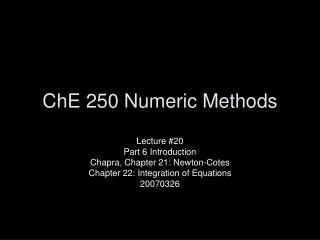DownloadDownload PresentationChE 250 Numeric Methods

# ChE 250 Numeric Methods

Download Presentation## ChE 250 Numeric Methods

- - - - - - - - - - - - - - - - - - - - - - - - - - - E N D - - - - - - - - - - - - - - - - - - - - - - - - - - -
##### Presentation Transcript

1. ChE 250 Numeric Methods Lecture #20 Part 6 Introduction Chapra, Chapter 21: Newton-Cotes Chapter 22: Integration of Equations 20070326

2. Class Schedule • Two weeks of lecture left • This week, Chapter 21-23 • Next week, Chapter 24-25 • We need to schedule your final exam • Cumulative chapters 1 through 22 • Choices: 11th, 13th,…other? • Project 2 • Due April 20th • Should be posted on course webpage next weekend

3. Today’s Lecture • Introduction • What integration and differentiation is used for • Chapter 21: Newton-Cotes Integration • Trapezoid Rule • Simpson’s 1/3 Rule • Simpson’s 3/8 Rule • Composite Methods • Chapter 22: Integration of Equations • Romberg Integration

4. Numeric Integration & Differentiation • Many times, it is not efficient to analytically derive the solution to an integral problem so numeric methods are used • If analyzing a data set, two approaches are available: • you can use interval methods like the trapezoidal rule or Simpson’s • you can use techniques discussed in part 5 to fit the data to a curve, then use calculus to calculate the definite integral

5. Numeric Integration & Differentiation • If the derivative is needed there are finite difference methods that can be used • Finite difference works for data or functions • it is preferred to use a curve instead of raw so that error does not propagate

6. Newton-Cotes Integration Formulas • For data or a function, the easy way to integrate is to approximate the values in the range of interest with a polynomial, then integrate the polynomial • The trapezoidal method uses first-order polynomial (straight lines) to connect adjacent points

7. Trapezoidal Rule • For a function that can be evaluated throughout the range from x0 to xn, where n is the number of equal base trapezoids, we can calculate the area:

8. Trapezoidal Rule • The error of approximating a curve with a straight line can be large • To reduce error, we can increase the number of intervals, however, round off error will eventually dominate, so a reasonable number for n is 100-200 • Example 21.2 • Questions?

9. Simpson’s Rules • For greater accuracy, each interval could be estimated using a higher order polynomial instead of a straight line Simpson’s 3/8 Simpson’s 1/3

10. Simpson’s 1/3 Rule • Approximating the curve with a second order Lagrange polynomial

11. Simpson’s 1/3 Rule • So, this will break n points into n/2 even base parabolic intervals (n must be even) • Example 21.5 • Questions?

12. Simpson’s 3/8 Rule • If a third order Lagrange interpolating polynomial is used, 4 data points are used for each interval • Because the accuracy is not much higher than the 1/3 rule and more points calculations are required, typically 3/8 is not used for the entire interval

13. Multiple ‘Rules’ • For a set of data with odd intervals, one set of four points is used with the 3/8 Rule and the remaining even number of intervals use 1/3 Rule • This method becomes more important with unevenly spaced intervals

14. Newton-Cotes Formulas • The formulas are summarized on page 604 along with the truncation error estimates • Example 21.7, 21.8

15. Integration of Equations • Romberg Integration is based on the trapezoidal rule, but it uses a recursive approach to refine the integral estimate quickly based on the values calculated by successive n=2j trapezoidal rule estimates

16. Integration of Equations • This method uses a call to a trapaziod subroutine to generate estimates • The estimates are then combined to form improved estimates with lower error n-1 times

17. Integration of Equations • This process is repeated until the approximation error between successive iterations falls below a stopping criterion • Example 22.10 • Questions?

18. Preparation for 28Mar • Reading • Chapter 22: Integration of Equations • Chapter 23: Numeric Differentiation • Homework: Due Friday April 6 • Chapter 21 • 9, 13, 20 • Chapter 22 • 3, 7, 14 • Chapter 23 • 1, 9, 12, 13, 14, 27 • Chapter 24 • 4, 5, 12